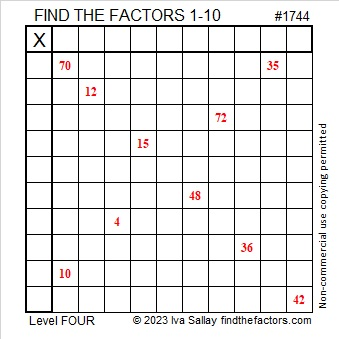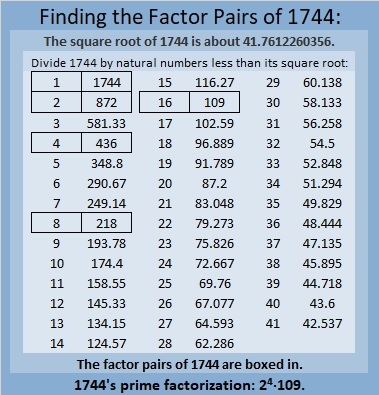# 1744 XOXO Kisses or Multiplication? XOXO

Contents

### Today’s Puzzle:

When I was a little girl, my mother told me that OXOXOX stands for Hugs and Kisses. That would mean that O means “hug” and X means “kiss”, but I think X also means multiplication. It’s almost Valentine’s Day, so in today’s puzzle, it can mean either one.

This is a Level-4 puzzle so the clues don’t come in any particular order. Use logic to place all the numbers 1 to 10 in the first column and in the top row.### Factors of 1744:

• 1744 is a composite number.
• Prime factorization: 1744 = 2 × 2 × 2 × 2 × 109, which can be written 1744 = 2⁴ × 109.
• 1744 has at least one exponent greater than 1 in its prime factorization so √1744 can be simplified. Taking the factor pair from the factor pair table below with the largest square number factor, we get √1744 = (√16)(√109) = 4√109.
• The exponents in the prime factorization are 4 and 1. Adding one to each exponent and multiplying we get (4 + 1)(1 + 1) = 5 × 2 = 10. Therefore 1744 has exactly 10 factors.
• The factors of 1744 are outlined with their factor pair partners in the graphic below.### More About the Number 1744:

1744 is the difference of two squares in three ways:
437² – 435² =1744,
220² – 216² =1744, and
113² – 105² =1744.

1744 is also the sum of two squares:
40² + 12² = 1744.

1744 is the hypotenuse of a Pythagorean triple:
960-1456-1744, calculated from 2(40)(12), 40² – 12², 40² + 12².
It is also 16 times (60-91-109).

This site uses Akismet to reduce spam. Learn how your comment data is processed.